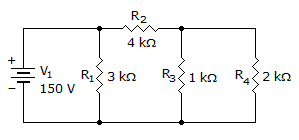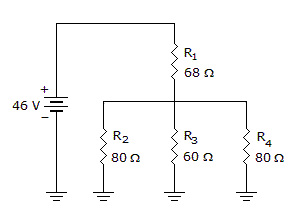# Electronics - Series-Parallel Circuits

### Exercise :: Series-Parallel Circuits - True or False

16.

The total power is 10 watts.A. True B. False

Explanation:

No answer description available for this question. Let us discuss.

17.The total resistance in the given circuit can be found using the formula:

RT = R1 + R2 || R3 || R4.

 A. True B. False

Explanation:

No answer description available for this question. Let us discuss.

18.

When a load resistance is connected to the output of a voltage divider circuit, the source current increases.

 A. True B. False

Explanation:

No answer description available for this question. Let us discuss.

19.

Opens and shorts are typical problems that occur in electric circuits.

 A. True B. False

Explanation:

No answer description available for this question. Let us discuss.

20.

A Wheatstone bridge may be used to determine the value of an unknown resistance.

 A. True B. False